# RS Aggarwal Class 10 Solutions Chapter 12 Circles

## RS Aggarwal Class 10 Chapter 12 Circles Solutions Free PDF

A closed shape without any line segments is called a Circle and it is important for students to understand the terminology associated with a Circle. Some of the various terms used are Arc, Tangent, Radius, Diameter, Semicircle, Circumference, etc. Understanding the area enclosed, equations, tangent lines and the circumference of length are all important concepts. It was initially a geometric shape conceptualized by the Greek mathematicians.

## Download PDF of RS Aggarwal Class 10 Chapter 12 – Circles

The RS Aggarwal Maths solutions of maths provided in the table below have been formulated in accordance with the latest syllabus of CBSE, which makes these solutions highly effective from exam point of view. It helps students to tackle difficult questions with ease.

Q. 1: Find the length of the tangent drawn to a circle with radius 8cm from a point 17cm away from the center of the circle.

Sol:

PA is the tangent to the circle with center O and radius AO = 8 cm. The point P is at a distance of 17 cm from O.

In $\Delta$ PAO, A = 90o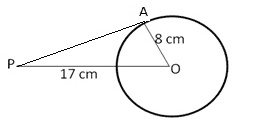By Pythagoras theorem:

PO2 = PA2 + AO2

Or PA2 = PO2 – AO2=> PA = $\sqrt{(17)^{2}-(8)^{2}}$ cm

= $\sqrt{289-64}$ cm

= $\sqrt{225}$ cm = 15 cm

Hence, the length of the tangent is 15 cm.

Q.2: A point P is 25cm away from the center of a circle and the length of the tangent drawn from P to the circle is 24cm. Find the radius of the circle.

Sol:

PA is the tangent to the circle with center O and radius, such that PO = 25 cm, PA = 24cm

In PAO, A = 90o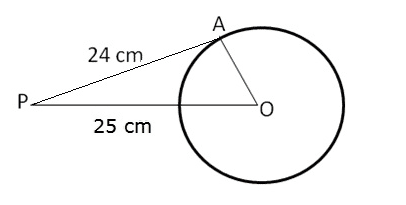By Pythagoras theorem:

PO2 = PA2 + AO2

Or OA2 = PO2 – PA2

=> OA = (25) 2 – (24) 2 cm

= (25 + 24) (25-24) cm =49 cm

Therefore, OA = 7 cm.

Hence, the radius of the circle is 7 cm.

Q. 3: Two concentric circles are the radii 6.5cm and 2.5cm. Find the length of the chord of the larger circle which touches the smaller circle.

Sol:

Given AP is a tangent at A and OA is radius through A and PA and PB are the tangent segments to circle with center O.

Therefore, OA is perpendicular to AP, similarly, OB is perpendicular to BP.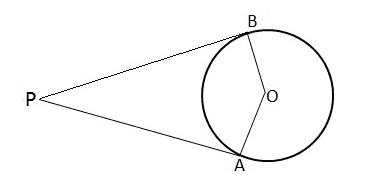Therefore, $\angle$ OAP = 90o

And $\angle$ OBP = 90o

So, $\angle$ OAP = $\angle$ OBP = 90o

Therefore, $\angle$OBP + $\angle$OAP = (90o + 90o) = 180o

Thus, the sum of opposite angles of quad. AOBP is 180o

Hence, AOBP is a cyclic quadrilateral.

Q. 4: In the given figure, a circle inscribed in a triangle ABC, touches the sides AB, BC, and AC at points D, E and F respectively. If AB = 12cm, BC = 8cm and AC = 10cm, find the lengths of AD, BE and CF.

Sol:

Given: From an external point P, tangent PA and PB are drawn to a circle with center O. CD is the tangent to the circle at a point E and PA = 14 cm.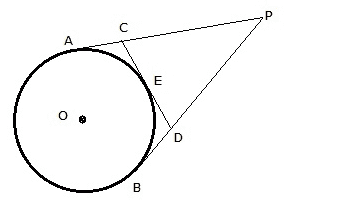Since the tangents from an external point are equal, we have PA = PB,

Also, CA = CE and DB = DE

Perimeter of $\Delta$ PCD = PC + CD + PD

= (PA – CA) + (CE + DE) + (PB – DB)

= (PA – CE) + (CE + DE) + (PB – DE)

= (PA + PB) = 2PA = (2 x 14) cm = 28 cm.

Hence, Perimeter of $\Delta$ PCD = 28 cm.

Q. 5: In the given figure, PA and PB are the tangents to a circle with center O. Show that the points A, O, B and P are concyclic.

Sol:

A circle is inscribed in a triangle ABC touching AB, BC and CA at P, Q and R respectively.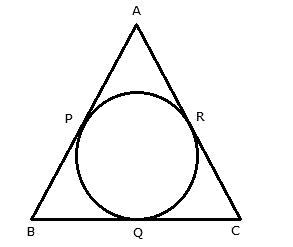Also, AB = 10 cm, AR = 7 cm, CR = 5 cm

AR and AP are the tangents to the circle.

AP = AR = 7cm

AB = 10 cm

BP = AB – AP = (10 – 7) = 3 cm

Also, BP and BQ are tangents to the circle.

BP = BQ = 3 cm

Further, CQ and CR are tangents to the circle.

CQ = CR = 5 cm.

BC = BQ + CQ = (3 + 5) cm = 8 cm.

Hence, BC = 8 cm

Q. 6: In the given figure, the chord AB of the larger of the two concentric circles, with center O, touches the smaller circle at C. Prove that AC = CB.

Sol:

Let the circle touches the sides AB, BC, CD, and DA at P, Q, R, S respectively.

We know that the lengths of tangents drawn from an exterior point to a circle are equal.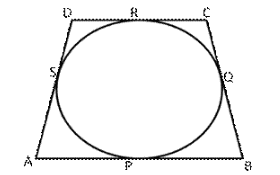AP = AS – – – – – – – – – – (1) {tangents from A}

BP = BQ – – – – – – – – – – (2) {tangents from B}

CR = CQ – – – – – – – – – – – (3) {tangents from C}

DR = DS – – – – – – – – – – – -(4) {tangents from D}

Adding (1), (2) and (3) we get:

AP + BP + CR + DR = AS + BQ + CQ + DS

=> (AP + BP) + (CR + DR) = (AS + DS) + (BQ + CQ)

=> AB + CD = AD + BC

=> AD = (AB + CD) – BC = {(6+4) – 7} cm = 3 cm

Q.7: From an external point P, tangents PA and PB are drawn to a circle with center O. If CD is the tangent to the circle at a point E and PA = 14cm, find the perimeter of ⧍PCD.

Sol:

Given: Two tangents segments BC and BD are drawn to a circle with center O such that  $\angle$CBD = 120o

Join OB, OC, and OD.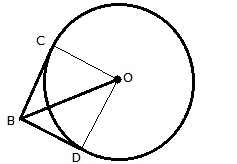In triangle OBC,

$\angle$ OBC = $\angle$ OBD = 60o

$\angle$ = 90o (BC is tangent to the circle)

Therefore, $\angle$ BOC = 30o

$\frac{BC}{OB}$ = sin 30o = $\frac{1}{2}$

=> OB = 2BC

Q. 8: A circle is inscribed in a ⧍ABC touching AB, BC and AC at P, Q and R respectively. If AB = 10cm, AR = 7cm and CR = 5cm, find the length of BC.

Sol:

Given O is the center of two concentric circles of radii 4 cm and 6 cm respectively. PA and PB are tangents to the outer and inner circle respectively. PA = 10 cm. Join OA, OB, and OP.

Then, OB = 4 cm, OA = 6 cm, PA = 10 cm.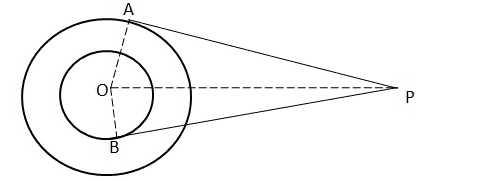In triangle OAP,

OP2 = OA2 + PA2 = (6)2 + (10)2 = 136 cm2

In $\Delta$ OBP

BP = $\sqrt{OP^{2}-OB^{2}}=\sqrt{136-16}$ cm = $\sqrt{120}$ cm = 10.9 cm.

Hence, BP = 10.9 cm

Q. 9: In the given figure, a circle touches all the four sides of a quadrilateral ABCD whose three sides are AB = 6cm, BC = 7cm and CD = 4cm. Find AD.

Sol: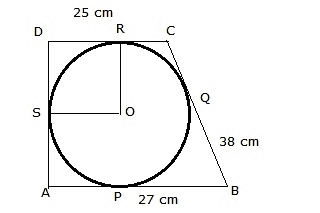Join OR and OS, then OR = OS

OR $\perp$ DR and OS $\perp$ DS

Therefore, ORDS is a square.

Tangents from an external point being equal, we have

BP = BQ

CQ = CR

DR = DS

Therefore, BQ = BP = 27 cm

=> BC – CQ = 27 cm

=> 38 – CQ = 27

=> CQ = 11 cm

=> CR = 11 cm

=> CD – DR = 11 cm

=> 25 – DR = 11 cm

=> DR = 14 cm

=> r = 14 cm

Q.10: In the given figure, an isosceles triangle ABC, with AB = AC, circumscribes a a circle. Prove that the point of contact P bisects the base BC

Sol:

Here, OA=OB and OA is perpendicular to AP,

OA is perpendicular to BP (Since tangents drawn from an external point are perpendicular to the radius at the point of contact)

∠OAP = 900, ∠OBP = 900

∠OAP+∠OBP=900+900=1 800

∠A0B+∠APB=1 800 (Since, ∠OAP+∠OBP+∠AOB+∠APB = 3600)

Sum of opposite angle of a quadrilateral is 180°.

Hence, A, O, B and P are concyclic.

Q.11: In the given figure, O is the center of two concentric circles of radii 4cm and 6cm respectively. PA and PB are tangents to the outer and inner circle respectively. If PA = 10cm, find the length of PB up to one place of decimal.

Sol: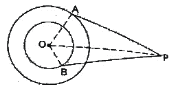We know that tangent segments to a circle from the same external point are congruent.

Now, we have

AR = AQ, BR = BP and CP = CQ

Now, AB = AC

=> AR + RB = AQ + QC

=> AR + RB = AR + QC

=> RB = QC

=> BP = CP

Hence, P bisects BC at P.

Q.12: In the given figure, O is the center of two concentric circles of radii 4cm and 6cm respectively. PA and PB are tangents to the outer and inner circle respectively. If PA = 10cm, find the length of PB up to one place of decimal.

Sol:

Construction: Join OA, OB, OC, OE perpendicular to AB at E and OF perpendicular to AC at F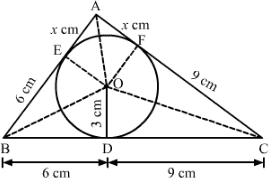We know that tangent segments to a circle from the same external point are congruent.

Now, we have,

AE = AF, BD = BE = 6 cm and CD = CF = 9 cm

Now,

Area ⧍ABC=Area ⧍BOC + Area ⧍AOB + Area ⧍AOC =>54

= 12 x BC x OD + 12xABx0E + 12 x AC x OF =>108

=>36=30+2x => x=3 cm

AB = 6 + 3 = 9cm

AC = 9 +3 = 12 cm.

Q.13: PQ is a chord of length 4.8cm of a circle of radius 3cm. The tangents at P and Q intersect at a point T as shown in the figure. Find the length of TP.

Sol: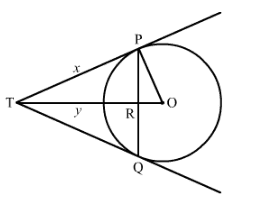Let TR = y and TP = x

We know that the perpendicular drawn from the center to the chord bisects it.

PR = RQ

Now, PR + RQ = 4.8

=>PR + PR = 4.8

=> PR = 2.4

Now, in right triangle POR:

By Using Pythagoras theorem, we have

PO2 = OR2 + PR2

=> 32 = OR2 + (2.4)2

OR2 = 3.24

OR = 1.8

Now, in right triangle TPR

By Using Pythagoras theorem, we have:

TP2 = TR2 + PR2 + x2 = y2 + (2.4) 2

x2 = y2 + 5.76  ……..(1)

Again, in right triangle TPQ

By Using Pythagoras theorem, we have:

TO2 = TP2 + PO2

(y + 1.8) 2 = x2 + 32

y2 + 3.6y + 3.24 = x2 + 9

y2 + 3.6y = x2 + 5.76 ……..(2)

Solving (1) and (2), we get:

x = 4 cm and y = 3.2 cm

TP = 4cm.

Q.14: Prove that the line segment joining the points of contact of two parallel tangents of a circle passes through its center.

Sol: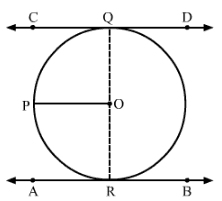Suppose CD and AB are two parallel tangents of a circle with center O

Construction:

Draw a line parallel to CD passing through O i.e, OP

We know that the radius and tangent are perpendicular at their point of contact. ∠OQC = ∠ORA = 90°

Now, ∠OQC + ∠POQ = 180° (co-interior angles)

=> ∠POQ = 180° – 90° = 90°

Similarly, Now, ∠ORA + ∠POR = 180° (co-interior angles)

∠POR = 180° – 90° = 90°

Now, ∠POR + ∠POO = 90° + 90° = 180°

Since ∠POR and ∠POQ are linear pair angles whose sum is 180°

Hence, QR is a straight line passing through center O.

Q.15: In the given figure, a circle with center O, is inscribed in a quadrilateral ABCD such that it touches the side BC, AB, AD and CD at points P, Q, R, S respectively. If AB = 29cm, AD = 23cm, ∠B = 90° and DS = 5cm then find the radius of the circle.

Sol: We know that tangent segments to a circle from the same external point are congruent.

Now, we have

DS = DR, AR = AQ

=> AR + RD = 23

=> AR = 23 – RD

=> AR=23- 5

=> AR = 18 cm

Again, AB = 29 cm

=> AQ+Q6=29

=> QB=29 -AQ

=> QB = 29 – 18

=> QB = 11 cm

Since all the angles are in a quadrilateral BQOP are right angles and OP = BQ. Hence, BQOP is a square.

We know that all the sides of the square are equal.

Therefore, BQ = PO = 11cm

Hence, the radius of the circle is 11 cm.

### RS Aggarwal Class 10 Solutions Chapter 12 – Circles

The RS Aggarwal Class 10 solutions for Chapter 12 are specifically designed to provide students with a detailed and accurate explanation for each and every question mentioned in the RS Aggarwal Class 10 maths textbook. The solutions are explained in an easy to understand way which enables the students to learn each and every concept quickly. Students are advised to practice the RS Aggarwal Class 10 solutions so that they are able to solve all the questions that will be asked in the final exam.

#### Practise This Question

A circle of diameter 6cm was drawn. From a point outside the circle, the length of the tangent drawn to the circle was found out to be 4cm. The distance between the point and the centre of the circle is ___.